NameFlagsCard.TypeDescription & Constraints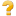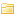Extension ..1Indigenous status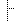url "http://hl7.org.au/fhir/StructureDefinition/indigenous-status"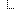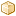valueCoding 1..CodingIndigenous Status Code
Binding: https://healthterminologies.gov.au/fhir/ValueSet/australian-indigenous-status-1 (required)Documentation for this format
<status value="draft"/> <date value="2018-10-03T00:00:00+10:00"/> <publisher value="Health Level Seven Australia (Patient Administration WG)"/> <contact> <telecom> <system value="url"/> <value value="http://hl7.org.au"/> <use value="work"/> </telecom> </contact> <description value="This extension applies to the Patient resource and provides a coding for Australian indigenous status of the patient."/> <purpose value="Recording an indigenous status for an Australian patient."/> <fhirVersion value="3.0.1"/> <mapping> <identity value="rim"/> <uri value="http://hl7.org/v3"/> <name value="RIM Mapping"/> </mapping> <kind value="complex-type"/> <abstract value="false"/> <contextType value="resource"/> <context value="Patient"/> <type value="Extension"/> <baseDefinition value="http://hl7.org/fhir/StructureDefinition/Extension"/> <derivation value="constraint"/> <snapshot> <element id="Extension"> <path value="Extension"/> <short value="Indigenous status"/> <definition value="National Health Data Dictionary (NHDD) based indigenous status for a patient."/> <min value="0"/> <max value="1"/> <base> <path value="Extension"/> <min value="0"/> <max value="*"/> </base> <condition value="ele-1"/> <constraint> <key value="ele-1"/> <severity value="error"/> <human value="All FHIR elements must have a @value or children"/> <expression value="hasValue() | (children().count() > id.count())"/> <xpath value="@value|f:*|h:div"/> <source value="Element"/> </constraint> <constraint> <key value="ext-1"/> <severity value="error"/> <human value="Must have either extensions or value[x], not both"/> <expression value="extension.exists() != value.exists()"/> <xpath value="exists(f:extension)!=exists(f:*[starts-with(local-name(.), 'value')])"/> <source value="Extension"/> </constraint> </element> <element id="Extension.id"> <path value="Extension.id"/> <representation value="xmlAttr"/> <short value="xml:id (or equivalent in JSON)"/> <definition value="unique id for the element within a resource (for internal references). This may be any string value that does not contain spaces."/> <min value="0"/> <max value="1"/> <base> <path value="Element.id"/> <min value="0"/> <max value="1"/> </base> <type> <code value="string"/> </type> <mapping> <identity value="rim"/> <map value="n/a"/> </mapping> </element> <element id="Extension.extension"> <path value="Extension.extension"/> <slicing> <discriminator> <type value="value"/> <path value="url"/> </discriminator> <description value="Extensions are always sliced by (at least) url"/> <rules value="open"/> </slicing> <short value="Additional Content defined by implementations"/> <definition value="May be used to represent additional information that is not part of the basic definition of the element. In order to make the use of extensions safe and manageable, there is a strict set of governance applied to the definition and use of extensions. Though any implementer is allowed to define an extension, there is a set of requirements that SHALL be met as part of the definition of the extension."/> <comment value="There can be no stigma associated with the use of extensions by any application, project, or standard - regardless of the institution or jurisdiction that uses or defines the extensions. The use of extensions is what allows the FHIR specification to retain a core level of simplicity for everyone."/> <alias value="extensions"/> <alias value="user content"/> <min value="0"/> <max value="*"/> <base> <path value="Element.extension"/> <min value="0"/> <max value="*"/> </base> <type> <code value="Extension"/> </type> <mapping> <identity value="rim"/> <map value="n/a"/> </mapping> </element> <element id="Extension.url"> <path value="Extension.url"/> <representation value="xmlAttr"/> <short value="identifies the meaning of the extension"/> <definition value="Source of the definition for the extension code - a logical name or a URL."/> <comment value="The definition may point directly to a computable or human-readable definition of the extensibility codes, or it may be a logical URI as declared in some other specification. The definition SHALL be a URI for the Structure Definition defining the extension."/> <min value="1"/> <max value="1"/> <base> <path value="Extension.url"/> <min value="1"/> <max value="1"/> </base> <type> <code value="uri"/> </type> <fixedUri value="http://hl7.org.au/fhir/StructureDefinition/indigenous-status"/> <mapping> <identity value="rim"/> <map value="N/A"/> </mapping> </element> <element id="Extension.valueCoding:valueCoding"> <path value="Extension.valueCoding"/> <sliceName value="valueCoding"/> <short value="Indigenous Status Code"/> <definition value="NHDD based indigenous status code."/> <min value="1"/> <max value="1"/> <base> <path value="Extension.value[x]"/> <min value="0"/> <max value="1"/> </base> <type> <code value="Coding"/> </type> <binding> <strength value="required"/> <description value="Indigenous Status"/> <valueSetReference> <reference value="https://healthterminologies.gov.au/fhir/ValueSet/australian-indigenous-status-1"/> </valueSetReference> </binding> <mapping> <identity value="rim"/> <map value="N/A"/> </mapping> </element> </snapshot> <differential> <element id="Extension"> <path value="Extension"/> <short value="Indigenous status"/> <definition value="National Health Data Dictionary (NHDD) based indigenous status for a patient."/> <max value="1"/> </element> <element id="Extension.url"> <path value="Extension.url"/> <fixedUri value="http://hl7.org.au/fhir/StructureDefinition/indigenous-status"/> </element> <element id="Extension.valueCoding:valueCoding"> <path value="Extension.valueCoding"/> <sliceName value="valueCoding"/> <short value="Indigenous Status Code"/> <definition value="NHDD based indigenous status code."/> <min value="1"/> <type> <code value="Coding"/> </type> <binding> <strength value="required"/> <description value="Indigenous Status"/> <valueSetReference> <reference value="https://healthterminologies.gov.au/fhir/ValueSet/australian-indigenous-status-1"/> </valueSetReference> </binding> </element> </differential> </StructureDefinition>